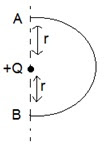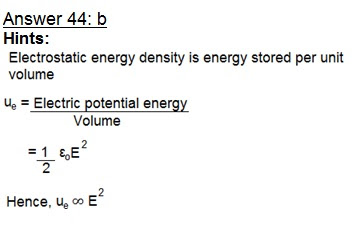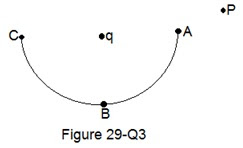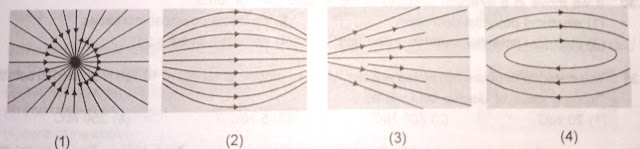## Services

Showing posts with label Electrostatics. Show all posts
Showing posts with label Electrostatics. Show all posts## Electrostatics : Physics Multiple Choice Questions with Hints and Solutions for JEE, NEET, MCAT

Electrostatics

https://www.indiastudysolution.com

### Physics Multiple Choice Questions with Hints and Solutions for preparation : Engineering (JEE), Medical (NEET), MCAT (Medical College Admission Test)

Hi Guys,

Welcome back to your own site India Study Solution @www.indiastudysolution.com

Here is your next Test Series a set of 10 most important solved questions (Q. No. 41-50) for NEET, MCAT and JEE from Electrostatics in Physics with their hints & solutions.

Click to check NEET and JEE Main Syllabus for chapter Electrostatics.

Friends if you have not subscribed us yet then you are missing many important things. Plz do subscribe India Study Solution (free, Subscribe button given in the sidebar) before you forget so that you get information through mail in your inbox whenever we publish anything of your interest.

You are also welcome to follow / share us on Face Book, Twitter.

Alternatively, you can Bookmark this site for timely updates on India Study Solutions, JEE and NEET syllabus, Notification (NTA circular etc.) posted in our EduNews section.

So, friends without wasting further time let us start -

### Electrostatics  India Study Solution Test Series – Set 5 (Q. No 41-50)

Question 41: Two electrons separated by distance ‘r’ experience a force F between them. The force between a proton and a singly ionized helium atom separated by distance 2r is

a. 4F

b. 2F

c. F/2

d. F/4

Question 42: A positive point charge Q is kept (as shown in the figure) inside a neutral conducting shell whose centre is at C. An external uniform electric field E is applied. Then:a. Force on Q due to E is zero

b. Net force on Q is zero

c. Net force acting on Q and conducting shell considered as a system is zero

d. Net force acting on the shell due to E is zero.

Question 43: Electric potential at a point P, r distance away due to a point charge q at point A is V. If twice of this charge is distributed uniformly on the surface of a hollow sphere of radius 4 r with centre at point A, the potential at point P now is

a. V

b. V/2

c. V/4

d. V/8

Question 44: If E is the electric field intensity of an electrostatic field, then the electrostatic energy density is proportional to:

a. E

b. E2

c. 1/E2

d. E3

Question 45: An α-particle is accelerated from rest through a potential of V volts. Its final kinetic energy is

a. 2 eV

b. 1 eV

c. 4 eV

d. ½ eV

Question 46: When a body is earth connected, electrons from the earth flow into the body. This means the body is initially

a. Unchanged

b. Charged positively

c. Charged negatively

d. An insulator

Question 47: An object is charged with positive charge. The potential at that object will be -

a. positive only

b. negative only

c. zero always

d. may be positive, negative or zero

Question 48: An electron enters into high potential region V2 from lower potential region V1 then its velocity

a. Will increase

b. Will change in direction of field

c. No change in direction of field

d. No change in direction perpendicular to field

Question 49: The work done in slowly moving an electron of charge e and mass m from A to B along a semi-circular path (as shown in the figure) in vertical plane in the field of charge Q isa. -2 mgr

b. –Qe/r

c. 2mgr + 2Qe/r

d. Zero

Question 50: Four identical charges +50 μC each are placed, one at each corner of a square of side 2m. How much external energy is required to bring another charge of +50 μC from infinity to the centre of the square?

a. 64 J

b. 41 J

c. 16 J

d. 10 J

India Study Solution Test Series
electrostatics
(Prepare for JEE, NEET, MCAT)
Hints and Solutions of Physics Electrostatics MCQ Test Series – Set 5 (Q. No.41–50)
https://www.indiastudysolution.com

(Hint: Force on Q due to E is QE and not zero. Force on Q is zero due to E & electric field of induced outer charges on sphere. Charge density on inner surface is not uniform therefore net force on charge Q is not zero. Due to E, net force on shell is zero because net charge on shell is zero.)Electrostatics: More practice questions
(Q.No. 21-30)                                     (Q.No. 51-60)  Next >>## NEET, JEE, MCAT Preparation | Solved Questions Bank, Test Series, Notes on Electrostatics, Physics chapters

Electrostatics

### India Study Solution MCQ Test Series with Hints and Solutions

JEE, NEET, MCAT (Medical College Admission Test)

Hi Guys,

Welcome back to your own site India Study Solution @www.indiastudysolution.com

Here is your next Test Series a set of 10 most important solved questions (Q. No. 31-40) for NEET, MCAT and JEE from Electrostatics in Physics with their hints & solutions.

Click to check NEET and JEE Main Syllabus for chapterElectrostatics.

Friends if you have not subscribed us yet then you are missing many important things. Plz do subscribe India Study Solution (free, Subscribe button given in the sidebar) before you forget so that you get information through mail in your inbox whenever we publish anything of your interest.

You are also welcome to follow / share us on Face Book, Twitter.

Alternatively, you can Bookmark this site for timely updates on India Study Solutions, JEE and NEET syllabus, Notification (NTA circular etc.) posted in our EduNews section.

So, friends without wasting further time let us start -

Electrostatics

India Study Solution Objective Questions Test Series
Set 4 (Q. No 31-40)

Question 31: Consider the situation of figure (29-Q3). The work done in taking a point charge from P to A is WA, from P to B is WB and from P to C is WC.a. WA< WB< WC

b. WA> WB> WC

c. WA = WB = WC

d. None of these

Question 32: When a glass rod is rubbed with silk, it:

a. gains electrons from silk

b. gives electrons to silk

c. gains protons from silk

d. gives protons to silk

Question 33: Two point charges of same magnitude and opposite sign are fixed at points A and B. A third small point charge is to be balanced at point P by the electrostatic force due to these two charges. The point P -a. lies on the perpendicular bisector of line AB

b. is at the mid-point of line AB

c. lies to the left of A

d. none of these

Question 34: In a certain region of space where electric potential is constant, the magnitude of electric field is

a. Also constant

b. Positive

c. Negative

d. Zero

Question 35: Relative permittivity of mica is:

a. one

b. less than one

c. more than one

d. infinite

Question 36: Potential due to a charged conducting shell varies as inversely proportional to the distance from its centre at

a. Inside the sphere

b. Outside the sphere

c. Only outside the sphere

d. Does not vary like mentioned

Question 37: The electric field above a uniformly charged non conducting sheet is E. If the non-conducting sheet is now replaced by a conducting sheet, with the charge same as before, the new electric field at the same point is:

a. 2E

b. E

c. E/2

d. None of these

Question 38: The wrong statement about electric lines of force is

a. These originate from positive charge and end on negative charge

b. They do not intersect each other at a point

c. They have the same form for a point charge and a sphere

d. They have a physical existence

Question 39: A negative charge is placed at some point on the line joining the two +Q charges at rest. The direction of motion of negative charge will depend upon the:

a. position of negative charge alone

b. magnitude of negative charge alone

c. both on the magnitude and position of negative charge

d. magnitude of positive charge

Question 40: If we move in direction opposite to the electric lines of force:

a. electrical potential decreases

b. electrical potential increases

c. electrical potential remains uncharged

d. nothing can be said

India Study Solution Test Series

electrostatics

(Prepare for JEE, NEET, MCAT)

Hints and Solutions of Physics Electrostatics MCQ Test Series – Set 4 (Q. No.31–40)

Answer 32: b (Hint: When we rub glass rod with silk, excess electrons are transferred from glass to silk. So, glass rod becomes positive and silk becomes negative).

Answer 33: d (Hint: There is no point near electric dipole having E = 0).Electrostatics: More practice questions

(Q.No. 21-30)                                     (Q.No. 41-50)  Next >>## NEET and JEE preparation | Physics Guide with Hints and Solutions | Electrostatics

Electrostatics

### India Study Solution MCQ Test Series

(Practice Questions with Hints and Solutions)

NEET, JEE Main, JEE Advanced | Medical and Engineering Entrance Exams | MBBS Admission Test

Hi Guys,

Welcome back to your own site India Study Solution @www.indiastudysolution.com

###Here is your next Test Series a set of 10 most important solved questions (Q. No. 21-30) for NEET and JEE from Electrostatics in Physics with their hints & solutions.

Click to check NEET and JEE Main Syllabus for chapter Electrostatics.

Friends if you have not subscribed us yet then please subscribe India Study Solution (it is free, Subscribe button given in the sidebar) before you forget so that you get information through mail in your inbox whenever we publish anything of your interest.

Bookmark our site for more updates on India Study Solutions, JEE Syllabus, NEET Syllabus and JEE and NEET related news (NTA circular etc.) in our EduNews section and of course, Physics Solutions and Guide.

Practice and prepare with the help of India Study Solution FREE online study materials to crack while preparing for NEET, JEE, IIT, AIIMS or any other Medical and Engineering Entrance Exams.

So, friends without wasting further time let us start -

Electrostatics  - Questions Bank with Solutions

India Study Solution Test Series – Set 3 (Q. No 21-30)

Question 21: When charge is given to a body

a. more charge accumulates at regions of small curvature

b. more charge accumulates at regions of large curvature

c. charge is distributed uniformly irrespective of curvature

d. none of the above is true

Question 22: Two equal positive charges are kept at points A and B. The electric potential at the points between A and B (excluding these points) is studied while moving from A to B. The potential

a. continuously increases

b. continuously decreases

c. increases then decreases

d. decreases then increases

Question 23: There are two metallic spheres of same radii but one is solid and the other is hollow, then:

a. solid sphere can be given more charge

b. hollow sphere can be given more charge

c. they can be charged equally (maximum)

d. none of the above

Question 24: A charge of 5C experiences a force of 5000N when it is kept in uniform electric field. What is the potential difference between two points separated by a distance of 1cm?

a. 10V

b. 250V

c. 1000V

d. 2500V

Question 25: The unit of permittivity of free space εo is

a. coulomb/newton-metre

b. coulomb2/newton-metre2

c. newton-metre2/coulomb2

d. coulomb2/(newton-metre)2

Question 26: The electric charge in uniform motion produces-

a. an electric field only

b. a magnetic field only

c. both electric and magnetic fields

d. neither electric nor magnetic fields

Question 27: A conductor has 14.4 x 10-19 C positive charge. The conductor has:

(Charge on electron = 1.6 x 10-19 C)

a. 9 electrons in excess

b. 27 electrons in short

c. 27 electrons in excess

d. 9 electrons in short

Question 28: An electric dipole is placed in a uniform electric field. The net electric force on the dipole

a. is always zero

b. depends on the orientation of the dipole

c. can never be zero

d. depends on the strength of the dipole

Question 29: A charged particle (charge q) is moving in a circle of radius R with uniform speed v. The associated magnetic moment μ is given by:

a. qvR/2

b. qvR2

c. qvr2/2

d. qvR

Question 30: The law, governing the force between electric charges is known as

a. Ampere’s law

b. Ohm’s law

d. Coulomb’s law

<><><><><><><><><><><><><>

India Study Solution - Test Series Answers

electrostatics

Hints and Solutions of Physics Electrostatics MCQ Test Series – Set 3 (Q. No.21–30)

(Hint: Both the spheres can be charged equally. Because for metallic sphere either solid or hollow, the charge will raised on the sphere. Since, both spheres have same surface area, so they can hold equal maximum charge)

Answer 27: d (Hint: As conductor has positive charge. So, there is deficiency of electrons).

(Hint: As revolving charge is equivalent to a current,
So,Electrostatics: Further study

(Q.No. 11-20)                                     (Q.No. 31-40)  Next >>## India Study Solution MCQ Test Series - Electrostatics | Physics practice questions for JEE, NEET

### India Study Solution Physics Test Series (Prepare and Practice)

NEET, JEE, IIT, AIIMS, NTSE | Engineering, BDS, MBBS Admission Test
Hi Guys,
Welcome back to your own site India Study Solution
Here is your next Set of Questions (Q. No. 11-20) for chapter Electrostatics from Physics with their hints & solutions (given at the end).
(Please scroll down to find Electrostatics syllabus for NEET and JEE).
This is a very important chapter and so, we would request you to please bookmark this site or if you have not subscribed us yet then please subscribe before you forget (it is free) so that you get information whenever we publish more questions on this and other chapter / subject.
Hope it would help you to learn and enhance your confidence while preparing for NEET, JEE (IIT), AIIMS, COMEDK, NEST or any other Medical and Engineering Entrance Exams.
So, friends without wasting time let us start -
Electrostatics
India Study Solution Test Series – Set 2 (Q. No 11-20)
Question 11: An electron is projected with certain velocity into an electric field in a direction opposite to the field. Then it is
a. accelerated
b. retarded
c. neither accelerated nor retarded
d. either accelerated or retarded

Question 12: If the electric potential at a point in free space is 5 x 1011 V, what will be the electric field at that point
a. 4πε0 x 1022 V/m
b. 12πε0 x 1020 V/m
c. 4πε0 x 1020 V/m
d. Nothing can be said

Question 13: In induction the charge induced in the near surface of a dielectric is
a. Equal and dissimilar
b. Greater and dissimilar
c. Lesser and dissimilar
d. Equal and similar

Question 14: Total electric force on an electric dipole placed in an electric field of a point charge is:
a. always zero
b. never zero
c. zero when mid-point of dipole coincides with the point charge
d. zero when dipole axis is along any electric line of force

Question 15: Which of the following is not the unit of charge?
b. coulomb
c. stat coulomb

Question 16: +2C and +6C two charge are repelling each other with a force of 12N. If each charge is given -2C of charge, the value of the force will be
a. 4N (Attractive)
b. 4N (Repulsive)
c. 8N (Repulsive)
d. Zero

Question 17: A point charge +Q is placed at the centroid of an equilateral triangle. When a second charge +Q is placed at a vertex of the triangle, the magnitude of the electrostatic force of the central charge is 8N. The magnitude of the net force on the central charge when a third charge +Q is placed at another vertex of the triangle is:
a. zero
b. 4N
c. 4√2 N
d. 8 N

Question 18: Two parallel plates carry opposite charges such that the electric field in the space between them is in upward direction. An electron is shot in the space and parallel to the plates. Its deflection from the original direction will be
a. Upwards
b. Downwards
c. Circular
d. Elliptical

Question 19: Angle between equipotential surface and lines of force is
a. Zero
b. 1800
c. 900
d. 450

Question 20: Consider the four field patterns shown. Assuming there are no changes in the regions shown, which of the patterns represents a possible electrostatic field:

a. 1
b. 2
c. 3
d. 4

Electrostatics (Syllabus for NEET and JEE): Introduction, Electric Charges and their properties, Frictional Electricity, Charges and their Conservation, Unit of Charge, Conductors and Insulators, Presence of free Charges and bound Charges inside a Conductor, Charging by Induction, Coulomb’s Law, Dielectric Constant,  Forces between Multiple Charges, Electric Field, Electric Field lines, Electric Flux, Electric Dipole, Dipole in a Uniform External Field, Torque on a Dipole in a uniform electric field,  Continuous Charges distribution, Gauss’s Law and its applications, Electrostatic Potential, Potential due to Point Charge / Electric dipole / System of charges, Equipotential Surfaces, Potential Energy of a System of Charges/ External Field,
India Study Solution Test Series
electrostatics
Hints and Solutions of Physics Electrostatics MCQ Test Series – Set 2 (Q. No.11–20)